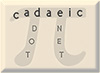Wild Narcissistic Numbers Mike Keith

In the most general sense, a narcissistic number is an integer that's equal to an expression involving its own digits. Often, this term is applied to the specific case of a number equal to a sum of powers of its digits, like the number 153:

153 = 1³ + 5³ + 3³

whic is equal to the sum of the cubes of its digits.

What I'm interested in here are wild narcissistic numbers, in which the expression for n (that also happens to be equal to n) still involves just the digits of n but in a more bizarre way. Here's a classic example:

362913 = 3!· 6 - F(2) + 9!· 1 - F(3)

where F(n) is the nth Fibonacci number [F(0) = 0, F(1) = 1, and F(n) = F(n-1) + F(n-2)]. The right hand side consists only of the digits in the number itself, in order (3, 6, 2, 9, 1, 3), but the expression involves addition, subtraction, multiplication, factorials, and Fibonacci numbers!

Just thinking about the number of different kinds of wild narcissistic numbers makes ones head hurt a little. Different mathematical operations could be used (add, subtract, multiply, divide, raise to a power, etc.) as well as various "unary functions" (factorial, subfactorial, etc.), binary functions (for example C(n,m) and P(n,m), the numbers of combinations and permutations of n things taken m at a time), and number sequences (like the nth Fibonacci or Catalan number). There are millions of different kinds, even for something as small as 6-digit numbers.

Well, I have only explored a tiny grain of sand in this large universe, but here are a few I've come up with.  The notation c(n) represents the nth Catalan number (in the sequence c(0)=1,1,2,5,14,42,132,429,1430,4862,...) and "¡" is the subfactorial function.

36 = 3!· 6
1448 = 1!· 4 + c(4) + c(8)
17812 = 17 + 8¡ + 12
24739 = 24 + 7! + 39
267499 = 26 + c(7) + 49 + c(9)

Here are some nice ones sent to me (Apr 2000) by Jean-Chearles Meyrignac:

71 = sqrt(7! + 1)
119 = -(1-({-(1 - sqrt(9)!)}!)
720 = (sqrt(7 + 2 + 0)!)!   and also, as pointed out to me by Amnon Melzer, = (7 - 20)!
733 = (7 + 3!) + (3!)!
936 = (sqrt(9)!)3 + 6!